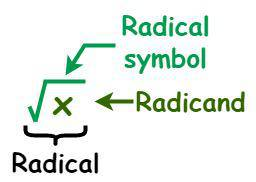GFG App
Open AppBrowser
Continue

The square root of any number is a value that gives the original number when multiplied by itself. The square root and square are inverse operations. For example, if a number “m” is the square root of a number “n”(m = √n), then the “n” is the square of “m” (n = m × m). We know that the square of any number is always positive, so every number has two square roots, where one is a positive value and the other is a negative value. For example, the value of the square root of 9 is equal to 3 and -3. The square root of 9 is denoted as √9, where 9 is called a radicand and “√” is called the radical symbol. The square root of a positive number x can be written as (x)1/2, where 1/2 is the exponent.  Now coming to the addition and subtraction of square roots, we can perform the operations just like we do with regular numbers. But remember that we can only add or subtract square roots or radicals that have the same radicand. In this article, let’s learn about the addition and subtraction of square roots in detail.Square root of an integer x

As we discussed above, we can only add or subtract square roots or radicals that have the same radicand. If two terms have the same radicand, then we can add or subtract their coefficients and leave the radicand as it is. The terms that have the same radicands are known as “like radicals”, whereas the terms that have different radicands are known as “unlike radicals.”

• First, simplify the given square roots if possible. So, try to factor them to find at least one perfect square factor.
• Once you have simplified the given square roots of the terms, find the like radicals.
• Finally, add or subtract the coefficients of like radicals and leave any additional terms as part of the equation.

Example: Solve 8√9 + 3√16.

Solution:

The given expression is 8√9 + 3√16

Here, both radicands are different. But we can simplify them further.

8√9 + 3√16 = 8√(32) + 3√(42)

= 8 × 3 + 3 × 4

= 24 + 12 = 36.

Thus, 8√9 + 3√16 = 36.

Also, Check

## Solved Examples

Example 1: Simplify: 5√8 + 3√32.

Solution:

Given expression: 5√8 + 3√32

5√(2×2×2) + 3√(2×24)

= 5 × 2√2 + 3 × 22√2

= 10√2 + 12√2

= 22√2

Hence, 5√8 + 3√32 = 22√2.

Example 2: Simplify 14√3 − 2√12.

Solution:

Given Expression: 14√3 − 2√12

14√3 − 2√12 = 14√3 − 2√(22×3)

= 14√3 − 2 ×2√3

= 14√3 − 4√3

= 10√3

Hence, 14√3 − 2√12 = 10√3.

Example 3: Solve: 7√(a2) − 2√(a4) + √(a2).

Solution:

Given Expression: 7√(a2) − 2√(a4) + √(a2)

= 7a − 2a2 + a

= 8a − 2a2

Thus, 7√(a2) − 2√(a4) + √(a2) = 8a − 2a2.

Example 4: Solve: 51√7 + 16√5 − 13√7 + 31√5.

Solution:

Given Expression: 51√7 + 16√5 − 13√7 + 31√5

= (51√7 − 13√7) + (16√5 + 31√5)

= 38√7 + 47√5

Thus, 51√7 + 16√5 − 13√7 + 31√5 = 38√7 + 47√5.

Example 5: Solve: 19√75 + 12√27 − 10√48.

Solution:

Given Expression: 19√75 + 12√27 − 10√48

= 19√(3 × 5 × 5) + 12√(3 × 3 × 3) − 10√(4 × 4 × 3)

= 19 × 5√3 + 12 × 3√3 − 10 × 4√3

= 95√3 + 36√3 − 40√3

= 91√3

Thus, 19√75 + 12√27 − 10√48 = 91√3.

Example 6: Simplify: 2√3 + 3√3.

Solution:

Given expression: 2√3 + 3√3

= 2√3 + 3√3

= 5√3

Hence, 2√3 + 3√3 = 5√3

Example 7: Simplify: √9 + √25.

Solution:

Given expression: √9 + √25

= √9 + √25

= 3 + 5

= 8

Hence, √9 + √25 = 8

Example 8: Simplify: √8 + 2√2

Solution:

Given expression: √8 + 2√2

= √8 + 2√2

= 2√2 + 2√2          ( As √8 = 2√2 )

= 4√2

Hence, √8 + 2√2 = 4√2

Example 9: Simplify: √3×(4√3 + 11 )

Solution:

Given expression: √3×(4√3 + 11 )

= √3×(4√3 + 11 )

= √3×4√3 + 11×√3

= 4×3 + 11×√3

= 12 + 11×√3

Example 10: Simplify: √5×(√5 + √6 )

Solution:

Given expression: √5×(√5 + √6 )

= √5×(√5 + √6 )

= √5×√5 + √5×√6

= 25 + √30

## FAQs on Square Roots

Question 1: What is a square root?

In mathematics, the square root of any number is a value that gives the original number when multiplied by itself. The square root and square are inverse operations. For example, if a number “m” is the square root of a number “n”(m = √n), then the “n” is the square of “m” (n = m × m).

Question 2: Can the square root be negative?

We know that the square of any number is always positive, so every number has two square roots, where one is a positive value and the other is a negative value. So, the square root of a number can be negative. For example, the value of the square root of 9 is equal to 3 and -3.

Question 3: What is the symbol of a square root?